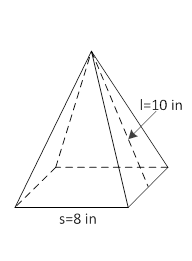### Sample Problem

The base of the following pyramid is a square. What is the volume of the pyramid? Round the answer to the nearest hundredth.V = in3.

#### Solutionh == 9.17 in

So, Volume of the pyramid

= 1/3 × 8 × 8 × 9.17

= 195.63 in3